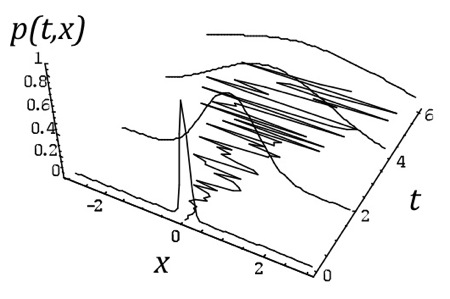/ #Fokker-Planck #Ito

# Itô calculus for the rest of us

One of the areas of my research is stochastic differential equations (SDE). I posted about it several times before. One of the things students and collaborators keep asking me about SDEs is the weird stochastic Itô Calculus. Itô Calculus is different from what you learn in 101 calculus. In particular, the chain rule is not longer valid. Let me explain it with an example. Suppose you have the following equation

$$dX = a dW(t)$$

where $$W(t)$$ is the Wiener stochastic process: it is a process in which $$W(0) = 0$$ and with Gaussian independent increments $$W(t)-W(s) \sim {\cal N}(0,t-s)$$, where $${\cal N}(\mu,\sigma^2)$$ are Gaussian random numbers with mean $$\mu$$ and variance $$\sigma^2$$. This equation is the simplest SDE and its solution is (obviously),

$$X(t) = a W(t)$$

But there is a different way to look at SDEs. Since they describe the trayectory of $$X(t)$$ subject to $$W(t)$$ we can instead ask ourselves what is the probability to find that $$X(t) = x$$ in average, i.e. after many different runs of $$W(t)$$. Let’s call $$P(x,t)$$that probability which is known to satisfy the Fokker-Planck equation

$$\frac{dP}{dt}=a\frac{d^2P}{dx^2}$$

which is the simple diffusion equation. The following figure shows the relationship between $$X(t)$$ and $$P(x,t)$$. While $$X(t)$$ is a simple trayectory (for a given $$W(t)$$ realization), $$P(x,t)$$ is the probability to find $$X(t) = x$$ over all possible realizations of $$W(t)$$.The relationship between $$X(t)$$ and $$P(x,t)$$ is more general than for the example used here. In general, if we have the SDE

$$dX(t) = f(X,t)dt + g(X,t) dW(t)$$

in the Itô sense, then $$P(x,t)$$ is the solution of

$$\frac{\partial P}{\partial t}= - \frac{\partial}{\partial x}\left[f(x,t)P(x,t)\right] + \frac{\partial^2}{\partial x^2}\left[g^2(x,t)P(x,t)\right]$$

The term $$f(x,t)$$ is usually know as the drift and $$g(x,t)$$ is the diffusion term.

Once the relationship between the Fokker-Planck equation and its SDE is given, it is very easy to understand Itô Calculus. For example, given the above SDE $$dX = a dW(t)$$, what is the SDE that will satisfy $$Y(t) = X^2(t)$$?

Instead of using Itô’s lemma,  we will use the Fokker Planck equation and traditional calculus. Thus $$\partial/\partial x = 2 \sqrt{y} \partial/\partial y$$. We also have that $$P(x,t) dx = P(y,t) dy$$ or $$P(x,t) = 2 \sqrt{y} P(y,t)$$. Putting all these things together we get

$$\frac{\partial}{\partial t}2\sqrt{y}P(y,t) = \frac{a^2}{2}\left\{2\frac{\partial}{\partial y}+4y\frac{\partial^2}{\partial y^2}\right\}2\sqrt{y} P(y,t)$$

and with a little bit of algebra we get

$$\frac{\partial P}{\partial t}=-\frac{\partial}{\partial y}[a^2 P(y,t)] + \frac{a^2}{2}\frac{\partial^2}{\partial y^2}[4yP(y,t)]$$

which, according to the general relationship between Fokker-Planck equation and SDE, corresponds to the following SDE

$$dY = a^2 dt + 2a \sqrt{Y} dW(t)$$

as the Itô’s lemma says. Obviously, this way to get the Itô lemma is painful in general, so you’d better use it directly on the SDE instead of the method shown here which goes through the Fokker-Planck equation. However, it is interesting to see that you can recover it from the Fokker-Planck equation.#### Esteban Moro

Professor at Universidad Carlos III de Madrid and MIT Medialab. Working on Complex Systems, Social Networks and Urban Science.